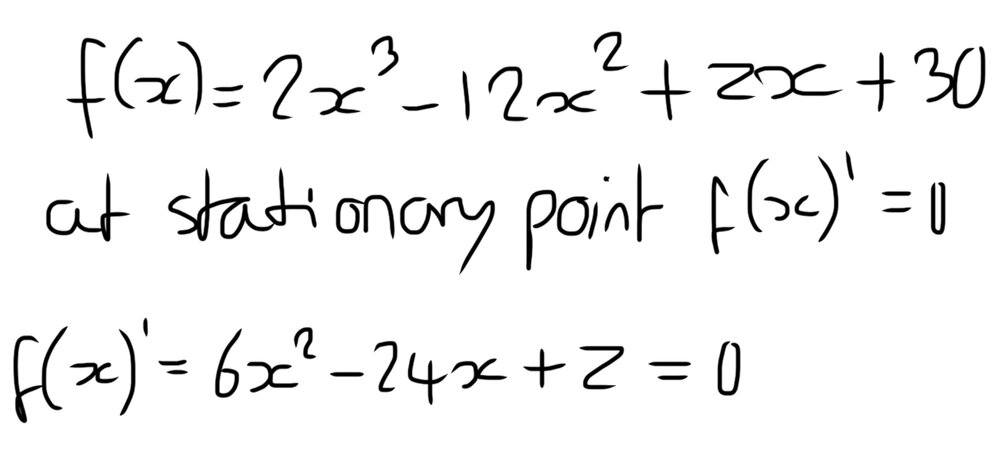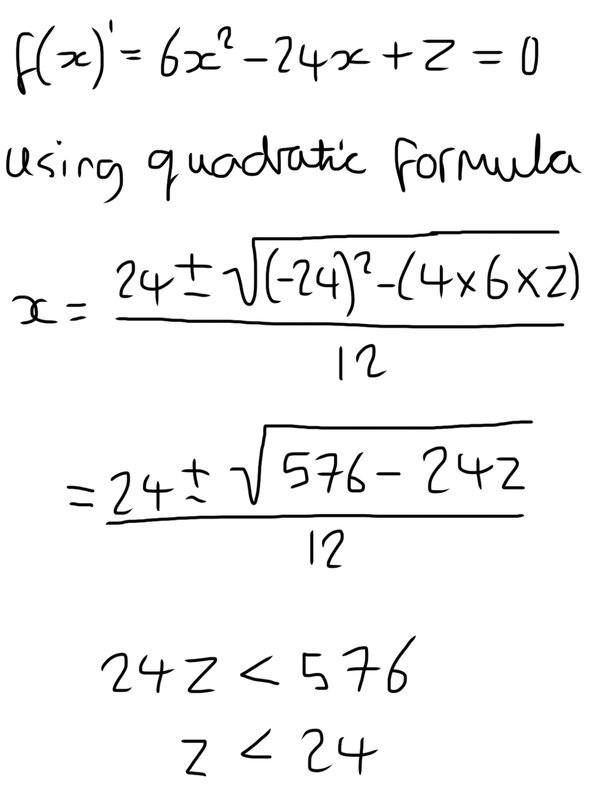# Value that gives more than one stationary point

maxim07
Homework Statement:
What value of z makes the curve have more than one stationary point
Relevant Equations:
f(x) = 2x^3 -12x^2 + zx + 30
Here is my attempt at a solutionI don’t know where to go from here, as the equation is a quadratic it will have two solutions which means there will be two stationary points, but I don’t know how to solve for z.

•Delta2

Homework Helper
Gold Member
2022 Award
I don’t know where to go from here, as the equation is a quadratic it will have two solutions
Does a quadratic always have two solutions?

•Delta2
maxim07
Not if both brackets are the same

Homework Helper
Gold Member
2022 Award
Not if both brackets are the same
I have no idea what you mean by that. What brackets?

••docnet and Delta2
Homework Helper
Gold Member
I don’t know where to go from here, as the equation is a quadratic it will have two solutions which means there will be two stationary points
That is only guaranteed if you allow complex solutions. When the problem expects only real solutions, the quadratic formula must have non-negative numbers inside the square root. That should allow you to get the restrictions on z.

•Delta2
maxim07
Not if both brackets are the same
I mean sometimes if you factor a quadratic the two bracketed terms you end up with are the same so you only have one solution

•Delta2
Homework Helper
Gold Member
2022 Award
I mean sometimes if you factor a quadratic the two bracketed terms you end up with are the same so you only have one solution

Homework Helper
Gold Member
I mean sometimes if you factor a quadratic the two bracketed terms you end up with are the same so you only have one solution
You need to use the quadratic formula. That will give you the factors, whether they are real, complex, integer, irrational, etc.

maxim07using the fact that the term under the square root can not be negative, this is what I get

•FactChecker and Delta2
Mentor
So to summarize, there will be two stationary points if z < 24.

What happens if z = 24? Or if z > 24?

Comment - the usual way of writing the derivative of f at a value x is f'(x), not f(x)'. IOW, the "prime" symbol (an apostrophe) goes immediately after the function name.

maxim07
If z = 24 you get sqrt(0) , if z>24 you get a negative in the square root, neither of which are possible

Mentor
If z = 24 you get sqrt(0) , if z>24 you get a negative in the square root, neither of which are possible
No, both these cases are possible. If z = 24, there is only one stationary point. If z > 24, there are no stationary points. In the latter case, this means that the graph of ##f(x) = 2x^3 - 12x^2 + zx + 30## is always increasing; i.e., has no points at which the tangent line is horizontal.

•docnet and FactChecker
maxim07
So if z =24 we get √0 so there is only one x solution and so one stationary point, if z > 24 we get √- so there are no solutions and no stationary points